Note: This is an archvied version of our old webpage. Some links might be broken. The current one can be found here.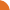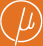Chair for Foundations of Software Reliability and Theoretical Computer Science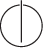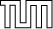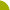Publications - Derivation Tree Analysis for Accelerated Fixed-Point ComputationReference:

Javier Esparza, Stefan Kiefer, and Michael Luttenberger. Derivation tree analysis for accelerated fixed-point computation. Technical report, Technische Universität München, Institut für Informatik, September 2008.

Abstract:

We show that for several classes of idempotent semirings the least fixed-point of a polynomial system of equations X = f(X) is equal to the least fixed-point of a linear system obtained by linearizing the polynomials of f in a certain way. Our proofs rely on derivation tree analysis, a proof principle that combines methods from algebra, calculus, and formal language theory, and was used to show that Newton's method over commutative and idempotent semirings converges in a linear number of steps. Our results lead to efficient generic algorithms for computing the least fixed-point. We use these algorithms to derive several consequences, including an O(N^3) algorithm for computing the throughput of a context-free grammar (obtained by speeding up the O(N^4) algorithm of Caucal et al.), and a generalization of Courcelle's result stating that the downward-closed image of a context-free language is regular.

Suggested BibTeX entry:

@techreport{EKL08:dltTechRep,
author = {Javier Esparza and Stefan Kiefer and Michael Luttenberger},
institution = {Technische Universit\"{a}t M\"{u}nchen, Institut f\"{u}r Informatik},
month = {September},
title = {Derivation Tree Analysis for Accelerated Fixed-Point Computation},
year = {2008}
}GZipped PostScript (174 kB)PDF (220 kB)Conference version